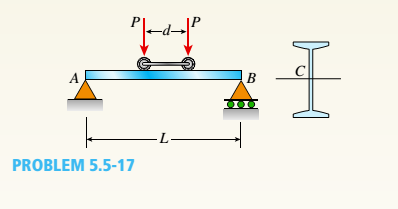# A simple beam A B of a span length L = 24 ft is subjected to two wheel loads acting at a distance d = 5 ft apart (see figure). Each wheel transmits a load P = 3.0 kips, and the carriage may occupy any position on the beam. Determine the maximum bending stress G m a x due to the wheel loads if the beam is an I-beam having section modulus S = 16.2 in 3 . If d = 5 ft. Find the required span length L to reduce the maximum stress in part (a) to 18 ksi. If L = 24 ft, Find the required wheel spacing s to reduce the maximum stress in part (a) to 18 ksi.### Mechanics of Materials (MindTap Co...

9th Edition
Barry J. Goodno + 1 other
Publisher: Cengage Learning
ISBN: 9781337093347
Chapter 5, Problem 5.5.17P
Textbook Problem
8 views

## A simple beam A B of a span length L = 24 ft is subjected to two wheel loads acting at a distance d = 5 ft apart (see figure). Each wheel transmits a load P = 3.0 kips, and the carriage may occupy any position on the beam. Determine the maximum bending stress Gmaxdue to the wheel loads if the beam is an I-beam having section modulus S = 16.2 in3. If d = 5 ft. Find the required span length L to reduce the maximum stress in part (a) to 18 ksi. If L = 24 ft, Find the required wheel spacing s to reduce the maximum stress in part (a) to 18 ksi.a.

To determine

The maximum bending stress due to wheel loads.

### Explanation of Solution

Given:

Length of beam, L = 24ft = 288 in. Distance between the wheel, d = 5ft = 60 in, Section modulus, S = 16.2 in., point load transmitted through one wheel, P = 3kip.

Concept Used:

The problem is carried out by using pure bending equation. When bending stress is developed along the axis of the material, the stress and strain can be calculated based on this equation

ER=MI=σyccR=σyE=εmax

E = young’s modulus, R = radius of gyration, M = Bending moment, I = moment of inertia, σy= normal stress, c = distance from the center to maximum stress.

Calculation:

Reaction a A

Ra=PL(2Ld2x)

Moment using Reaction at A, M=PL(2Lxdx2x

b.

To determine

The length required if the maximum stress is 18ksi

c.

To determine

The wheel spacing‘d’ for 18kip stress provided L = 24ft

### Still sussing out bartleby?

Check out a sample textbook solution.

See a sample solution

#### The Solution to Your Study Problems

Bartleby provides explanations to thousands of textbook problems written by our experts, many with advanced degrees!

Get Started

Find more solutions based on key concepts
A workpiece offset is the distance from __________ to _________.

Precision Machining Technology (MindTap Course List)

Which security protocols are predominantly used in Web-based e-commerce?

Principles of Information Security (MindTap Course List)

Give an example of a linear model in engineering.

Engineering Fundamentals: An Introduction to Engineering (MindTap Course List)

Determine the resultant of the force system shown.

International Edition---engineering Mechanics: Statics, 4th Edition

What is optoisolation?

Electric Motor Control

How do malware authors use social media to spread infection? (110)

Enhanced Discovering Computers 2017 (Shelly Cashman Series) (MindTap Course List)

If your motherboard supports ECC DDR3 memory, can you substitute non-ECC DDR3 memory?

A+ Guide to Hardware (Standalone Book) (MindTap Course List)